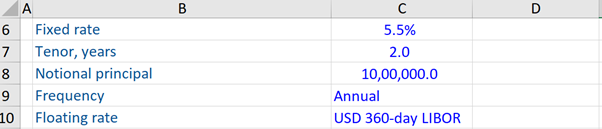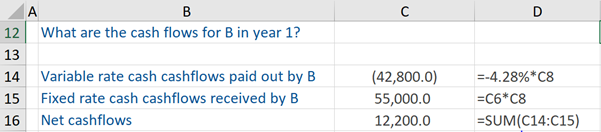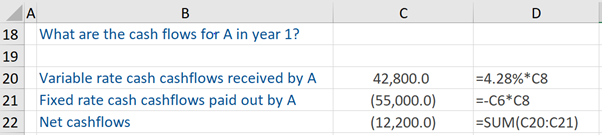## What are Financial Instruments?

Financial instruments (for example, bonds, securities, and cheques, etc.) are essentially monetary assets that are involved in contractual obligations between involved parties. This can be, for example, where one company agrees to pay cash for a bond and the other company is obligated to deliver the bond to them in exchange for the cash. These assets can be purchased, traded, created, or modified. Most types of such instruments can be viewed as packages of capital that may be traded – which enables the efficient flow of capital or its transfer between investors across the globe. Typically, there are three kinds of financial instruments – cash, foreign exchange, and derivative instruments.

## Key Learning Points

• Financial instruments are typical of three types – cash, foreign exchange, and derivatives instruments
• They can also be categorized according to asset classes – debt or equity-based financial instruments
• In the derivatives category, under the fixed income sub-category, a common type of derivative is the interest rate swap – here two parties simply swap fixed interest payments for floating-rate payments

## Types of Financial Instruments

Cash Instruments: these are financial instruments (securities, loans, and deposits) whose value can be determined directly from the markets and securities that can be transferred readily.

Foreign Exchange Instruments: these financial instruments are in the form of cash (spot exchange rate) and in the form of exchange-traded derivatives, currency swaps and futures, and foreign exchange options, and outright forwards.

Derivative Instruments: is a financial instrument whose value changes in response to the change in a specified interest rate, stock price, bond price, commodity price, foreign exchange rate, etc. Basically, the value of these types of instruments is determined from the underlying assets such as those mentioned above. Derivatives can either be exchange-traded or over-the-counter (OTC) derivatives.

There are four basic types of derivatives – Forward Contracts, Futures Contracts, Options Contracts, and Swaps. A forward contract is a customized contract between two parties to purchase or sell an asset at some specified price (decided in the present) at a future date.

Financial Instruments can also be categorized according to asset classes – such as debt-based financial instruments and equity-based financial instruments.

Examples of debt-based financial instruments are US Treasuries and commercial paper, deposits, certificates of deposit, mortgages, short-term interest futures, bonds, loans, bond futures, interest rate swaps, etc. Examples of equity-based financial instruments are common stocks, preferred stocks, convertible debentures, spot foreign exchange, currency futures, and swaps.

## Financial Instruments – Interest Rate Swap

A common kind of fixed income derivative is interest rate swaps.

In an interest rate swap, the parties concerned simply swap fixed interest payments for floating-rate payments (or floating rate for floating rate). The floating interest is defined typically as LIBOR interest. Other types of interest rate swaps include cross-currency and equity swaps.

Given below is a workout of a fixed for floating interest rate swap, to gain insight into the basic cash flows of such a swap.

At the outset, the first party (A) enters into an arrangement with the second party (B) i.e. a fixed for floating interest rate swap arrangement with the second party. The date on which this interest rate swap was created, the USD 360 day LIBOR was 4.28%. The terms of this arrangement are stated below. With reference to the tenor which is 2 years, this effectively means that the 2-year swap rate when this trade was done was 5.5%.We are told the cash flows for party B in the first year. So, we calculate the variable rate cash flows that are paid out by them (i.e. -4.28% * US\$1,000,000 (notional principal)). Thereafter, since party B is the receiver, we calculate the fixed-rate cash flows received by them. This is calculated as 5.5% * US\$ 1,000,000 (notional principal).

The net cash flows for party B are equal to the difference between these two calculations.If we look at the opposite side of the trade – we are interested in the cash flows for party A, which is the payer (of the fixed-rate) in the first year. This party will receive variable or floating rate cash flows, which is calculated as 4.28% * US\$1,000,000 (notional principal). But this payer will pay the fixed-rate cash flows, which is calculated as -5.5% * US\$ 1,000,000 (notional principal).

The net cash flows for party A are equal to the difference between these two computations. To conclude, the cash flows from the first and the second party is a mirror image of each other.Learn more about financial instruments by taking enrolling in our online private equity course – The Private Equity Associate.